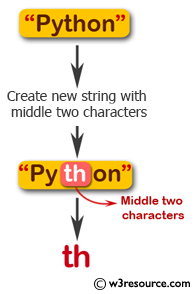﻿ Swift String Programming Exercise: Create a new string taking the middle two characters of a given string of even length - w3resource# Swift String Exercises: Create a new string taking the middle two characters of a given string of even length

## Swift String Programming: Exercise-12 with Solution

Write a Swift program to create a new string taking the middle two characters of a given string of even length. The given string length must be at least 2.

Pictorial Presentation:Sample Solution:

Swift Code:

``````import Foundation
func middleTwo(_ str: String) -> String {
var end_index = str.endIndex
var start_index = str.startIndex

while end_index != start_index {
start_index = str.index(after: start_index)
end_index = str.index(before: end_index)
}

end_index = str.index(after: end_index)
start_index = str.index(before: start_index)

return str.substring(with: Range(uncheckedBounds: (start_index, end_index)))
}
print(middleTwo("Python"))
print(middleTwo("Java"))
```
```

Sample Output:

```
th
av
```

Swift Programming Code Editor:

Improve this sample solution and post your code through Disqus

What is the difficulty level of this exercise?

﻿